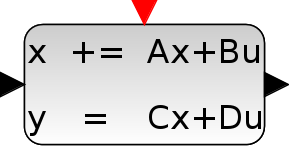# DLSS

Discrete state-space system

### Block Screenshot### Description

This block realizes a discrete-time linear state-space system. The system is defined by the matrices and the initial state . The dimensions must be compatible. At the arrival of an input event on the unique input event port, the state is updated.

### Parameters• A matrix

Square matrix.

Properties : Type 'mat' of size [-1,-1].

• B matrix

The B matrix. [] if system has no input.

Properties : Type 'mat' of size ["size(%1,2)","-1"].

• C matrix

The C matrix. [] if system has no output.

Properties : Type 'mat' of size ["-1","size(%1,2)"].

• D matrix

The D matrix. [] if system has no D term.

Properties : Type 'mat' of size [-1,-1].

• Initial state

A vector/scalar initial state of the system.

Properties : Type 'vec' of size "size(%1,2)".

### Default properties

• always active: no

• direct-feedthrough: no

• zero-crossing: no

• mode: no

• regular inputs:

- port 1 : size [1,1] / type 1

• regular outputs:

- port 1 : size [1,1] / type 1

• number/sizes of activation inputs: 1

• number/sizes of activation outputs: 0

• continuous-time state: no

• discrete-time state: yes

• object discrete-time state: no

• name of computational function: dsslti4

### Interfacing function

• SCI/modules/scicos_blocks/macros/Linear/DLSS.sci

### Computational function

• SCI/modules/scicos_blocks/src/c/dsslti4.c (Type 4)

 Report an issue << DLRADAPT_f Discrete time systems palette DOLLAR_f >>